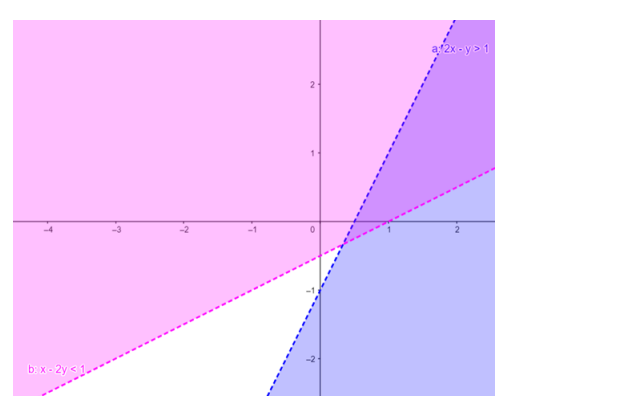# Solve the given inequalities

Question:

Solve the given inequalities $2 x-y>1, x-2 y<1$ graphically in two-dimensional plane.

Solution:

The graphical representation of $2 x-y>1, x-2 y<1$ is given by common region in the figure below.

$2 x-y>1 \ldots \ldots$ (1)

$x-2 y<1 \ldots \ldots(2)$

Inequality (1) represents the region below line $2 x-y=1$ (excluding the line $2 x-y=1$ ).

Inequality (2) represents the region above line $x-2 y=1$ (excluding the line $x-2 y=1$ ).

Therefore,every point in the common shaded region including the points on the respective lines represents the solution for the given inequalities.

This can be represented as follows,